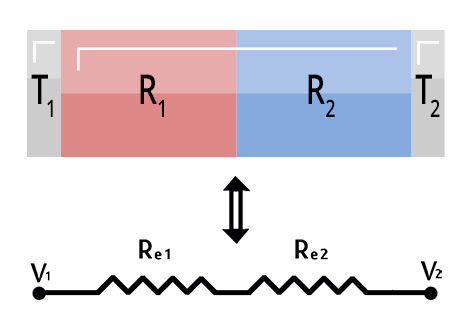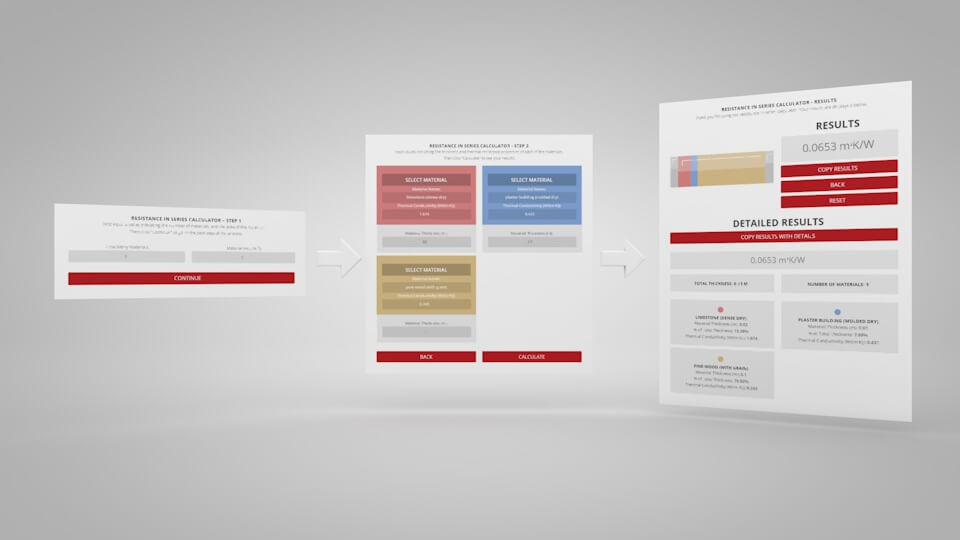Join us at the International Thermal Conductivity Conference (ITCC) and the International Thermal Expansion Symposium (ITES).

# Resistance in Series Calculator

How to calculate thermal resistance? The thermal resistance of a whole object (like a wall) can be calculated from the resistance of each individual layer. Choose however many layers your object has, then fill out the table with each layer’s thickness, area, and resistance. You can use the material database to find the values of specific materials. All layers should have the same area, but they can have different thicknesses. Units of the calculated thermal resistance are Kelvins per Watt (K/W), and the inputs should be in meters (m), meters squares () and meters Kelvin per Watt (mK/W).

Thermal Resistance:
$R = x / (A \ast k)$ Where:
$$R$$ = thermal resistance
$$x$$ = penetration depth or width of material
$$A$$ = area
$$k$$ = thermal conductivity#### Introducing the Thermal Resistance in Series Calculator

Want to learn more about thermal resistance in series calculator? Check out our blog post to learn how it works.

### Notes of interest:

1. This does not account for contact resistance between the different layers or components.
Effects like this should be directly measured.
2. Values for thickness (m) and area (m2) are entered manually. The material’s conductivity (W/mK) can be entered manually or selected from our materials database by clicking the SELECT MATERIAL button, then selecting a material from the left.

## RESISTANCE IN SERIES CALCULATOR – Step 1

First input a value indicating the number of materials, and the area of the materials.
Then click “Continue” to go to the next step of the process.

How Many Materials:
Material Area (m2):